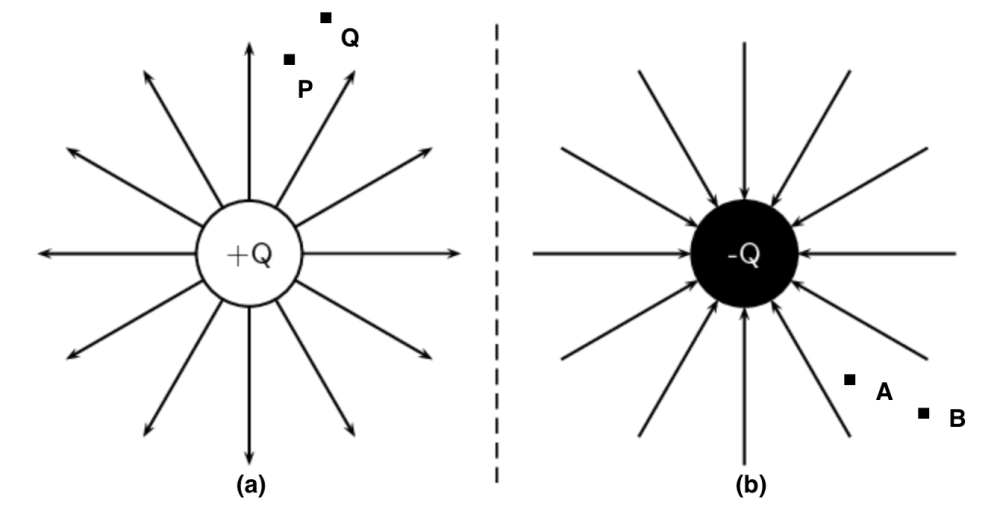Home/Class 12/Physics/

## QuestionPhysicsClass 12

Figures $$(a)$$and $$(b)$$ show the field lines of a positive and negative point charge respectively.Does the kinetic energy of a small negative charge increase or decrease in going from $$B$$  to $$A$$ ?

Due to force of repulsion on the negative charge, velocity decreases and hence the kinetic energy decreases in going from $$B$$  to $$A$$.